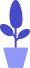# Python for loop

Estimated reading: 4 minutes 76 views

In addition to the while loop, there is a more readable loop structure, the for loop, which repeats the same piece of code a certain number of times. The for loop makes writing a loop much simpler: just create a variable, keep looping as long as the condition is true, and modify the variable at the end of each iteration of the loop.

## for loop

In Python, a for statement has the following parts:

• for keyword;
• variable;
• in keyword;
• Scope;
• colon;
• An indented block of code starting on the next line.

Tip: for loops are used to loop code a certain number of times, while while loops are repeated as long as a certain condition is met.A very common use of a for loop is to perform an operation on each element in a list, or on each character in a string (in terminology, this operation is called a traversal or iteration). For example, the for loop shown below will print out the animals in the zoo. The code is as follows.

``````animals = ["Tiger","Lion","Panda","Bear","Welf"]
for animals in animals:
print ("This zoo contains a "+animal+".")``````

The result of running this program is shown in Figure 1.

Figure 1
We can also use a for loop to rewrite the while loop that counted sheep earlier. The code is as follows.

``````for sheepCounted in [0,1,2,3,4,5,6,7,8,9]:
print("I have counted "+str(sheepCounted)+ " sheep.")
print("I fall asleep.")``````

The results obtained are shown in Figure 2.

Figure 2
As you can see, sheepCounted is a variable that is assigned to each value in the list in turn, and for each value, the block of statements is executed once. For the sake of simplicity, here we just count from 0 to 9 and fall asleep.

## range function

Instead of using a list to list every value that is used for every variable, we can do this with the help of the range function. The range function generates an arithmetic range array. For example, range(10) generates integers from 0 to 9. Note that if there is only one parameter, this parameter is the right boundary, and the left boundary defaults to 0.

For the above example, we can use range(10) to replace [0,1,2,3,4,5,6,7,8,9] with the following code.

``````for sheepCounted in range(10):
print("I have counted "+str(sheepCounted)+ " sheep.")
print("I fall asleep.")``````

The result obtained is the same, as shown in Figure 3.

Image 3
If you don’t want to start at 0, give the range function two arguments: a starting number and an ending number. Let’s take counting sheep as an example, this time give the range function two numbers, 1 and 31. Python will return a list of numbers, starting with the first number (1) and ending with the second number minus 1 (ie 31-1). The code is as follows

``````for sheepCounted in range(1,31):
print("I have counted "+str(sheepCounted)+ " sheep.")
print("I fall asleep.")``````

The result is to count from 1 to 30, and then fall asleep, as shown in Figure 4.

Figure 4
We can also make the range() function increment by a certain value. In this case, we need to enter 3 parameters, namely: start number, end number and increment. Taking the example of counting sheep again, if we just want to count even numbers, we tell range() to start at 2 and increase by 2 each time. Here, we give range() a starting number of 2, an ending number of 30 (31 minus 1) and an increment of 2. The code is as follows.

``````for sheepCounted in range(2,31,2):
print("I have counted "+str(sheepCounted)+ " sheep.")
print("I fall asleep.")``````

This time, the sheep counted are all even, as shown in Figure 5.